# AP Calculus BC Practice Test 14

### Test Information9 questions27 minutes

Calculator Allowed

1. Let y = f(x) be the solution to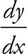= 2x - y with f(1) = 5. Use Euler’s method to approximate f(3), starting at x = 1 with a step size of 0.5.

2. If P(x) = x - 2x2 + 3x4 - 4x6 is the sixth degree Taylor polynomial for the function f about x = 0, what is f′′(0)?

3. The side of a square is decreasing at a constant rate of 0.4 cm/s. What is the rate of decrease of the perimeter of the square when the area of the square is 16 m2?

4.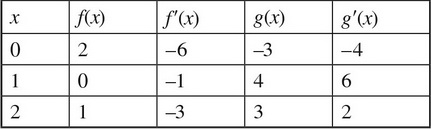In the table above, if h(x) = g(f(x)), then h′(2) =

5.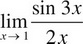=

6. Profits, in dollars, for a small business can be modeled by P(t) =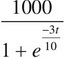, where t is measured in years. What are the total profits in years 5 ≤ t ≤ 8, to the nearest dollar?

7. The position of a particle at any time t ≥ 0 is given by x(t) = 4cos 2t and y(t) = 3sin 4t. What is the particle’s speed at time t = 5 seconds?

8. What is the minimum value of f(x) =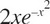on the closed interval [-1, 2]?

9. Which of the following integrals gives the area of the region enclosed by r = 3 - 2cos 4θ on the closed interval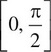?